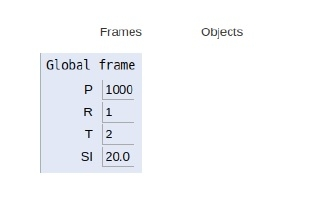# Python Program for simple interest

In this article, we will learn about the calculation of simple interest in Python 3.x. Or earlier.

Simple interest is calculated by multiplying the daily interest rate by the principal amount by the number of days that elapse between the payments.

Mathematically,

Simple Interest = (P x T x R)/100
Where,
P is the principal amount
T is the time and
R is the rate

For example,

If P = 1000,R = 1,T = 2
Then SI=20.0
Now let’s see how we can implement a simple interest calculator in
Python.

## Example

Live Demo

P = 1000
R = 1
T = 2
# simple interest
SI = (P * R * T) / 100
print("simple interest is", SI)

## Output

simple interest is 20.0

Here the simple interest is obtained three arithmetic products and one arithmetic division.

Now let’s see the scope of the variables declared. As shown in the figure below all variables are declared in the global scope.